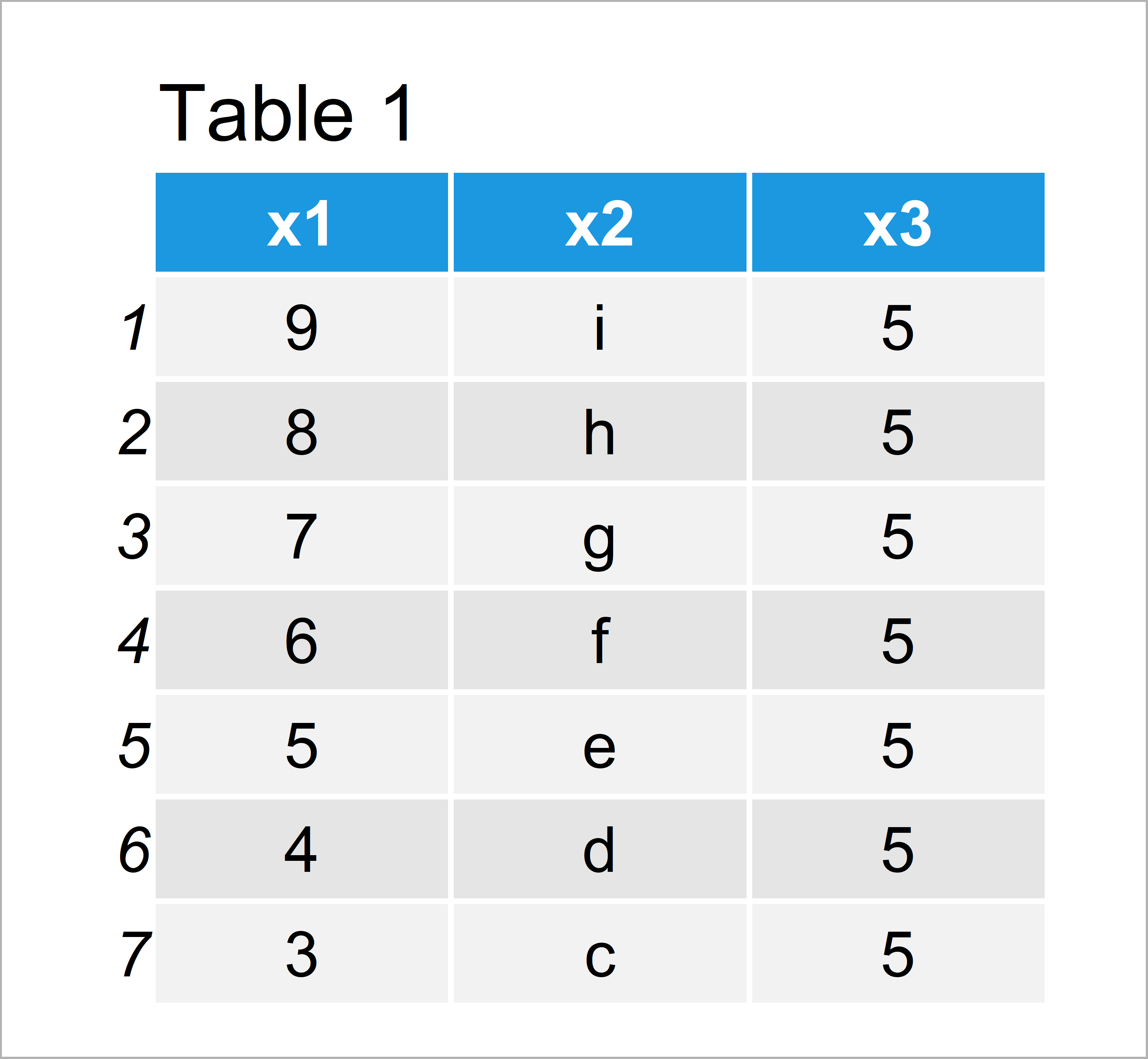# Extract Data Frame Rows that do not Match Logical Condition in R (Example)

In this R tutorial you’ll learn how to select data frame rows that have no match to a certain condition.

The content of the tutorial is structured as follows:

So now the part you have been waiting for – the exemplifying R code.

## Creation of Example Data

The data below will be used as basement for this R tutorial:

```data <- data.frame(x1 = 9:3,              # Creating data frame in R
x2 = letters[9:3],
x3 = 5)
data                                      # Printing data frame in R```Table 1 shows that our example data consists of seven rows and three columns.

Let’s also create a vector to which we will match a logical condition later on:

```vec <- c("d", "e", "g")                   # Creating vector in R
vec                                       # Printing vector in R
#  "d" "e" "g"```

Our example vector simply contains the character values “d”, “e”, and “g”.

## Example: Subset Data Frame Rows that do not Match Using ! and %in% Operators

In this section, I’ll illustrate how to extract only those data frame rows that do not match a certain logical condition.

More precisely, we’ll subset all rows where the values of the column x2 do not exist in our example vector vec.

For this, we can use the ! and \$ operators as shown below:

```data_new <- data[! data\$x2 %in% vec, ]    # Subsetting data frame
data_new                                  # Printing updated data frame in R```As you can see in Table 2, we have created a data frame without the three rows that have matched our logical condition.

## Video, Further Resources & Summary

Please accept YouTube cookies to play this video. By accepting you will be accessing content from YouTube, a service provided by an external third party.If you accept this notice, your choice will be saved and the page will refresh.

Furthermore, you might want to read the related tutorials which I have published on https://statisticsglobe.com/:

To summarize: In this article, I have shown how to keep only rows of a data frame that do not match a logical condition in R. Let me know in the comments below, in case you have further questions.

Subscribe to the Statistics Globe Newsletter